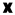# Number Master

The object of the game is to guess the hidden four-digit number (1023 to 9876). There are no duplicate digits in the number. Once you have guessed, a code will be shown that indicates how successful your guess was.= correct digit in the right place= correct digit in the wrong place= incorrect digit

Note: these do not tell you the correct position of any digit, just the respective counts of each.

 #11 8695= four correct, correct place #10 5968= four correct, wrong place #9 8652= two correct, correct place + one correct, wrong place #8 7421= none correct #7 7512= one correct, wrong place #6 9510= two correct, wrong place #5 5217= one correct, wrong place #4 0325= one correct, correct place #3 9652= one correct, correct place + two correct, wrong place #2 5678= one correct, correct place + two correct, wrong place #1 1234= none correct